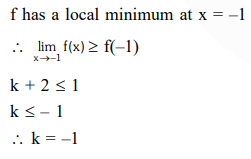# Solve this following`
Question:

Let $f: R \rightarrow R$ be defined by $f(x)=\left\{\begin{array}{lll}k-2 x, & \text { if } & x \leq-1 \\ 2 x+3, & \text { if } & x>-1\end{array}\right.$

If $\mathrm{f}$ has a local minimum at $x=-1$, then a possible value of $\mathrm{k}$ is :

1. 1

2. 0

3. $-\frac{1}{2}$

4. $-1$

Correct Option: , 4

Solution: